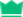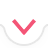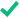# 导数

00:00:003 / 3
抱歉，您的浏览器不支持此应用
x^2
x^3
x^{\msquare}
\frac{\msquare}{\msquare}
\sqrt{\square}
\nthroot[\msquare]{\square}
\log_{\msquare}
\ln
\pi
\theta
\infty
\mathrm{发散}
正确答案 :)
让我们再次尝试 :(
尝试进一步化简
这个问题是否有帮助?
请告诉我们详情 »
感谢您！
主题
开始测验 00:00:00
上一问题
1 / 10
x^2
x^3
x^{\msquare}
\frac{\msquare}{\msquare}
\sqrt{\square}
\nthroot[\msquare]{\square}
\log_{\msquare}
\ln
\pi
\theta
\infty
\mathrm{发散}
Finish Quiz

Symbolab

# 速查表

Submit Assignment 重新开始 返回

# 速查表

再次开始 返回
Prev Student Next Student

01:12:13 小时返回
返回

## 步骤

抱歉，您的浏览器不支持此应用
编辑测验
测验已保存!您尚未获得任何皇冠

# 个测验

我没有账户

发送重置链接

### 我们已发送电子邮件至:

[email protected]
加入10000万快乐用户大家庭!
我已注册

继续访问网站 »

继续访问网站 »

### 交易失败!

请使用其他支付方法重试

订阅以获取更多信息:

•完整访问求解步骤
•网络和移动订阅
•笔记簿(无限存储)
•个性化的练习问题
•个测验
•详细的进度报告
•无广告
Remind me later »
 一次支付 $10.99 美元 2 个月 Weekly Subscription$1.99 美元/周 (直至取消) 月度订阅 $4.99 美元/月 (直至取消) 年度订阅$29.99 美元/年 (直至取消)
用户数据缺失
请联系支持

## 我们希望您能提供反馈

(可选)
(可选)

请添加消息。

已收到消息。感谢您的反馈。

### Generating PDF...一次支付 $10.99 美元 2 个月 Weekly Subscription$1.99 美元/周 (直至取消) 月度订阅 $4.99 美元/月 (直至取消) 年度订阅$29.99 美元/年 (直至取消)